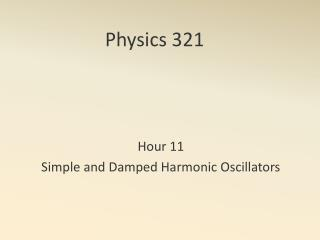DownloadDownload PresentationPhysics 321

# Physics 321

Download Presentation## Physics 321

- - - - - - - - - - - - - - - - - - - - - - - - - - - E N D - - - - - - - - - - - - - - - - - - - - - - - - - - -
##### Presentation Transcript

1. Physics 321 Hour 11 Simple and Damped Harmonic Oscillators

2. Find U and T Equilibrium Equation of motion ω, T Energy plot Mass on a Massless Spring y

3. y Assume Find U and T Equation of motion ω, T x A Shallow Frictionless Bowl z

4. y Assume Find U and T Newton’s Equations x Another Bowl z

5. Lissajous.nb Example

6. The equation: A little rearranging: Damped Oscillator A trial solution:

7. Underdamped: • Critically damped: • Overdamped: Three Regimes

8. Solution: or Underdamped

9. Solution: Overdamped

10. Solution: Critically Damped

11. DampOsc5_4.nb Example

12. Physics 321 Hour 12 Driven Harmonic Oscillators

13. The equation: Let The oscillator wants to oscillate at but the driver forces it to oscillate at . This leads to transient vs steady state behavior! Driven Oscillator

14. Driven_Osc.nb Example

15. We assume a solution something like But So we employ a trick… The driving force is the real part of Driven Oscillator

16. We assume a solution of the form This gives: Driven Oscillator

17. Conclusion 1: Driven Oscillator

18. Real parts: Imaginary parts: Driven Oscillator

19. Conclusion 2: And finally the steady state solution is: Driven Oscillator